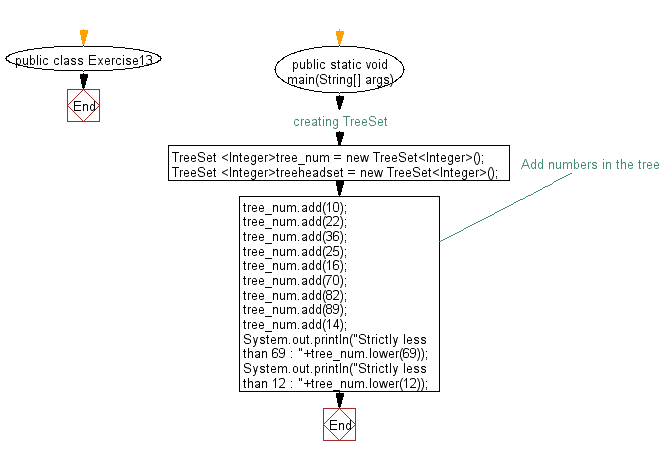﻿ Java Collection, TreeSet Exercises: Get an element in a tree set which is strictly less than the given element - w3resource

# Java Collection, TreeSet Exercises: Get an element in a tree set which is strictly less than the given element

## Java Collection, TreeSet Exercises: Exercise-13 with Solution

Write a Java program to get an element in a tree set which is strictly less than the given element.

Sample Solution:

Java Code:

``````import java.util.TreeSet;
import java.util.Iterator;

public class Exercise13 {
public static void main(String[] args) {
// creating TreeSet
TreeSet <Integer>tree_num = new TreeSet<Integer>();

// Add numbers in the tree

System.out.println("Strictly less than 69 : "+tree_num.lower(69));
System.out.println("Strictly less than 12 : "+tree_num.lower(12));
}
}
``````

Sample Output:

```Strictly less than 69 : 36
Strictly less than 12 : 10```

Flowchart:Java Code Editor: